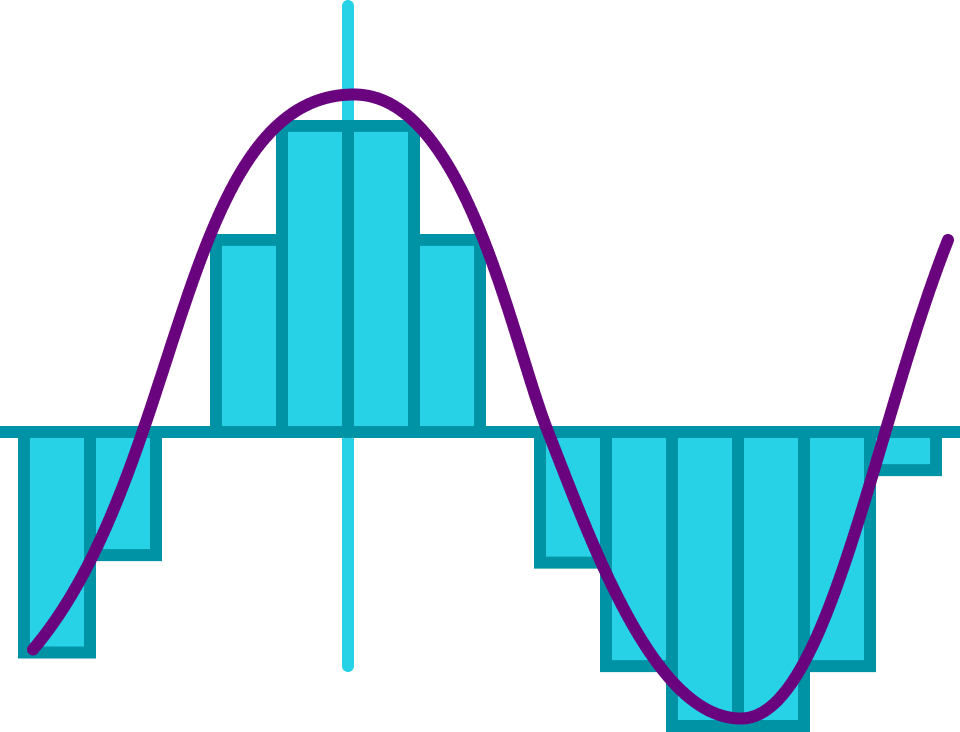# Zeno's Paradox

The opening unit showed us that calculus certainly has its practical aspects. Calculus can help us find the extreme values of a function and compute volumes, probabilities, and a host of other scientific quantities.

It comes as a bit of a pleasant surprise that calculus can also resolve ancient philosophical riddles like Zeno's paradox of motion. This unit will guide you to a basic understanding of the paradox and how it relates to infinite series. We'll spoil the ending by telling you how calculus resolves the paradox, but the details won't be set in place until the penultimate chapter of our course.

Only basic algebra is needed for this unit.

## Zeno's Paradox

### Introduction to Calculus

# Zeno's Paradox

Achilles is the “fleetest foot of all mortals”—meaning he's a pretty fast runner. One day, a snail named Zeno challenges him to a race. “Achilles,” says the snail, “I'll wager the Golden Fleece that I can beat you in a race if you but give me a head start.”

Achilles, knowing he would look pretty good in that Fleece, responds, “You’re on! How can I possibly lose?”## Zeno's Paradox

### Introduction to Calculus

# Zeno's Paradox

Achilles waits at the starting tree as Zeno makes his way to one half of the total 200-meter distance to the finish line at the grey stone.Let’s assume Achilles runs at a constant speed of 10 meters per second. We’ll also assume that Zeno runs at a rate of 1 meter per second, which is super fast for a snail!

After 10 seconds, where are Achilles and Zeno?

Note: Marks are measured away from the starting point, so the 90-meter mark is 90 meters from the start, for instance.

## Zeno's Paradox

### Introduction to Calculus

# Zeno's Paradox

10 seconds into the race, Achilles is at the 100-meter mark and Zeno is at 110 meters. At what meter mark will Zeno be when Achilles makes it to the 110-meter mark?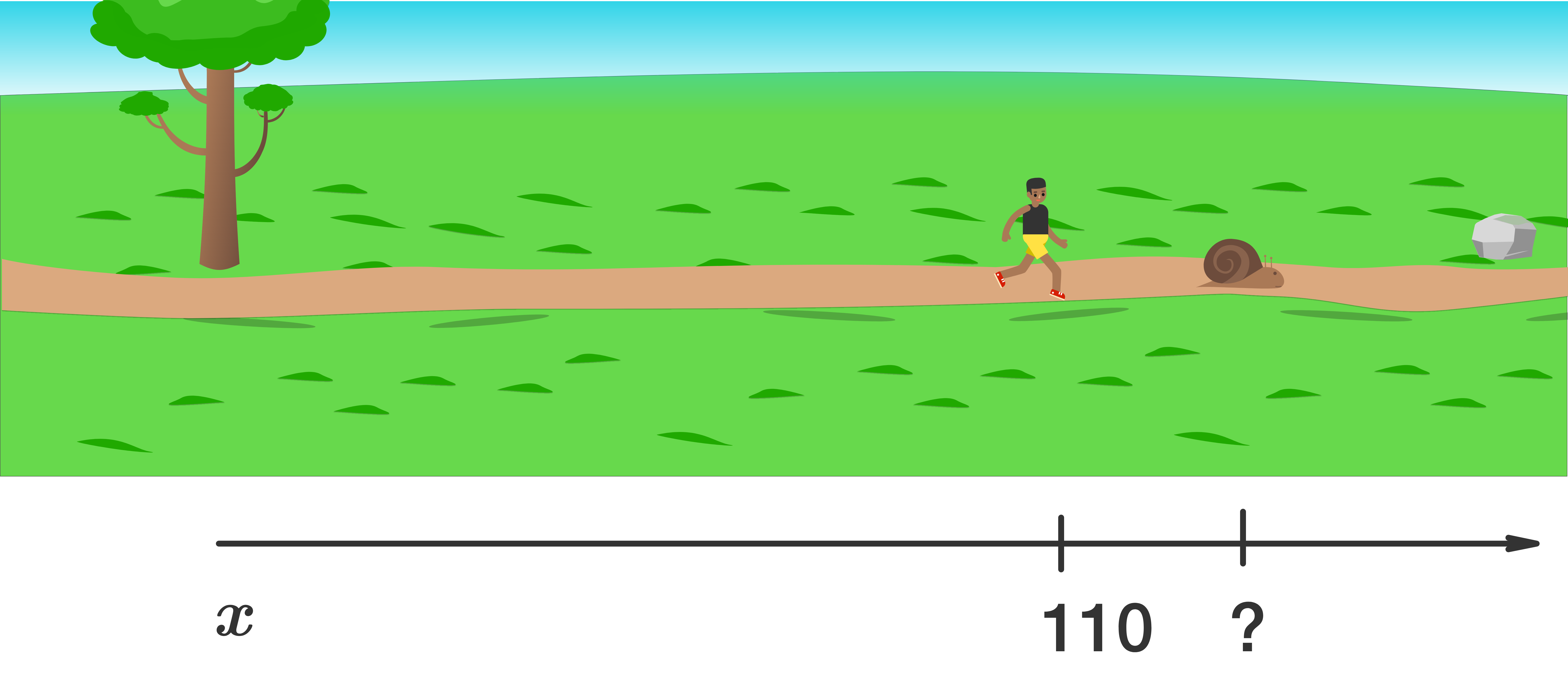## Zeno's Paradox

### Introduction to Calculus

# Zeno's Paradox

At this point in the race, Achilles starts to see why Zeno the snail was so certain he could win with a head start. By the time Achilles reaches a mark where Zeno was before, he still has farther to go.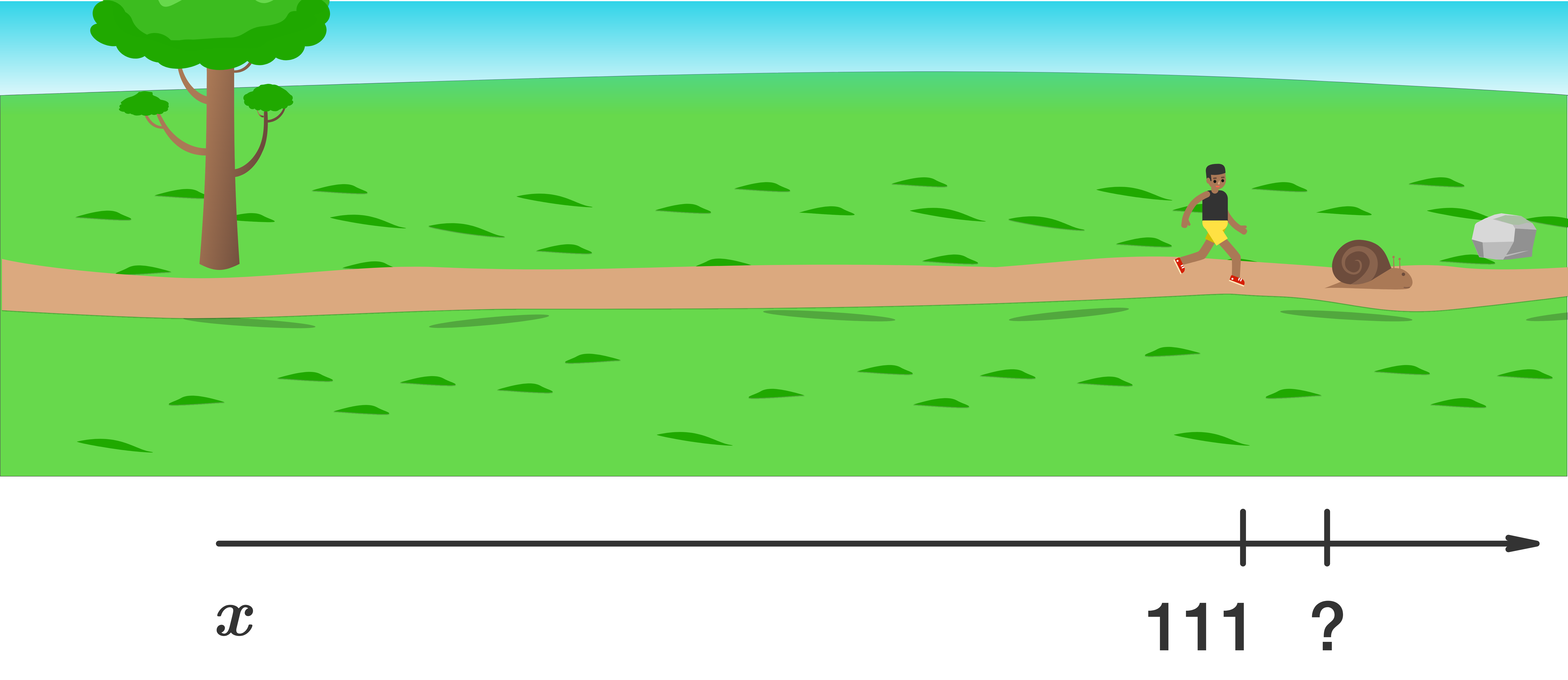When Achilles reaches the 111-meter mark, where is Zeno?

## Zeno's Paradox

### Introduction to Calculus

# Zeno's Paradox

It looks like the time it takes Achilles to catch up with Zeno is $$10+ 1 + 0.1 + 0.01 + \cdots$$ seconds, where the dots represent an infinite number of terms.

This problem comes from the Greek philosopher by the name of Zeno and is meant as a thought experiment. The heart of Zeno's paradox is the impossibility of completing an infinite sum. This seems perfectly reasonable. After all, no matter how fast you are, adding two numbers together takes some amount of time. So it seems like adding an infinite number of numbers should take an infinite amount of time!

It took the invention of calculus, in particular, infinite series, to put Zeno firmly to rest. We'll need much more machinery before we can show how calculus does this, but for the time being let's go ahead and figure out exactly how much time it takes Achilles to catch up to the snail.

## Zeno's Paradox

### Introduction to Calculus

# Zeno's Paradox

Earlier, we found $x_{A}(t) = \left( \SI[per-mode=symbol]{10}{\meter\per\second}\right) t \ , \ x_{Z}(t) = \left( \SI[per-mode=symbol]{1}{\meter\per\second}\right) t + \SI{100}{\metre}.$ Using these functions, where $$t$$ is in seconds, compute the time it takes for Achilles to reach Zeno. Enter your answer as a decimal.

## Zeno's Paradox

### Introduction to Calculus

# Zeno's Paradox

The resolution of Zeno's paradox comes from showing that $10 + 1 + 0.1 + 0.01 + \dots = 11.\bar{1} = \frac{100}{9}.$ In particular, we have to make sense of what it even means to sum an infinite number of numbers. This is precisely what the chapter on infinite series does and more.

There, we'll not only demonstrate this particular sum, but we'll also connect such infinite sums to topics like quantum theory, differential equations, and one of the most mysterious and useful formulas of all mathematics: Euler's formula.

The key notion underlying the entire chapter on infinite series (indeed, the entire course!) is the limit. In short, even though we can't add an infinite number of numbers, we can add a finite number of terms and then take the limit as the size of the sum goes to $$\infty.$$

## Zeno's Paradox

### Introduction to Calculus

# Zeno's Paradox

Infinite sums also play an integral role in solving another ancient mathematical riddle: How can we determine the area of a planar region bounded by a set of curves?

We learn how to compute the area of a region with straight edges (like a triangle or rectangle) early on, but regions with a curved edge like the one below (blue) are more challenging.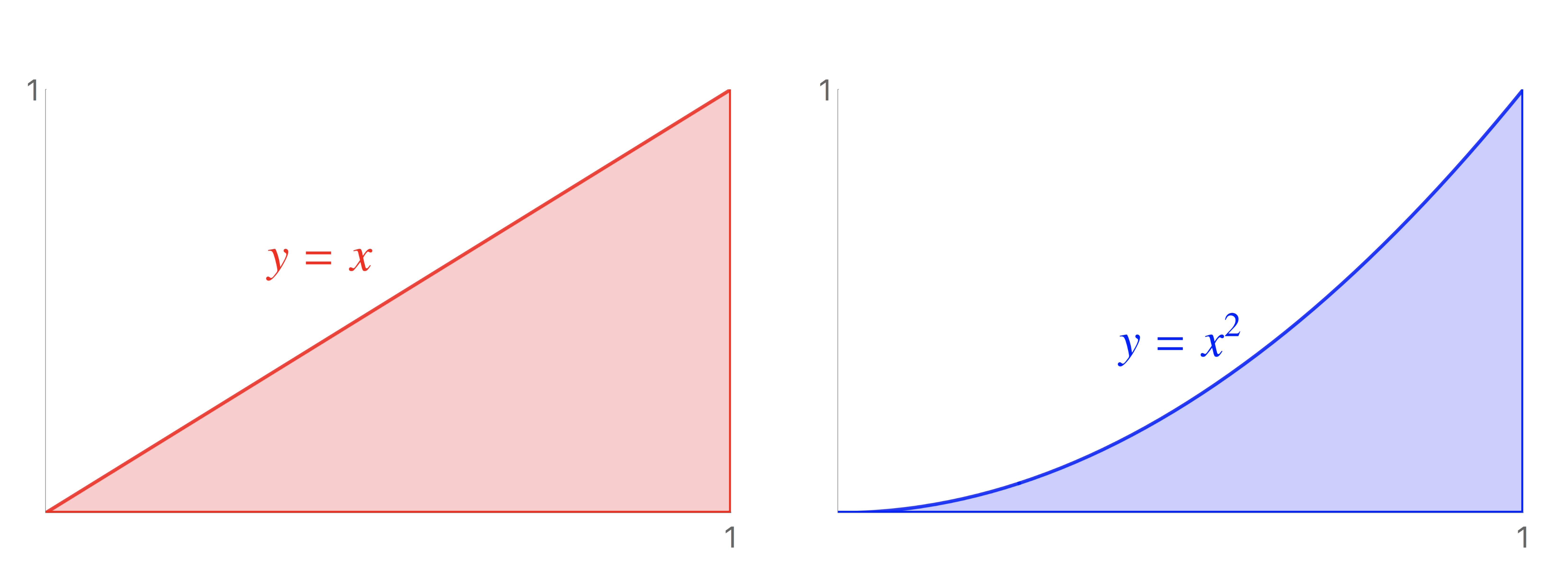The blue region bounded in part by the parabola $$y = x^2$$ looks similar to a right triangle with the hypotenuse bent inward. In fact, we can use such a triangle (red, left) to get an upper bound on the parabolic area: $\text{Blue area} \leq \frac{1}{2} ( \text{red base} ) ( \text{red height} ) = \frac{1}{2}.$ The calculus of infinite series allows us to go further and find the exact answer using straight-edge figures and a limit.

We can approximate the area below the parabola by a finite set of adjacent rectangles as in the figure below (left).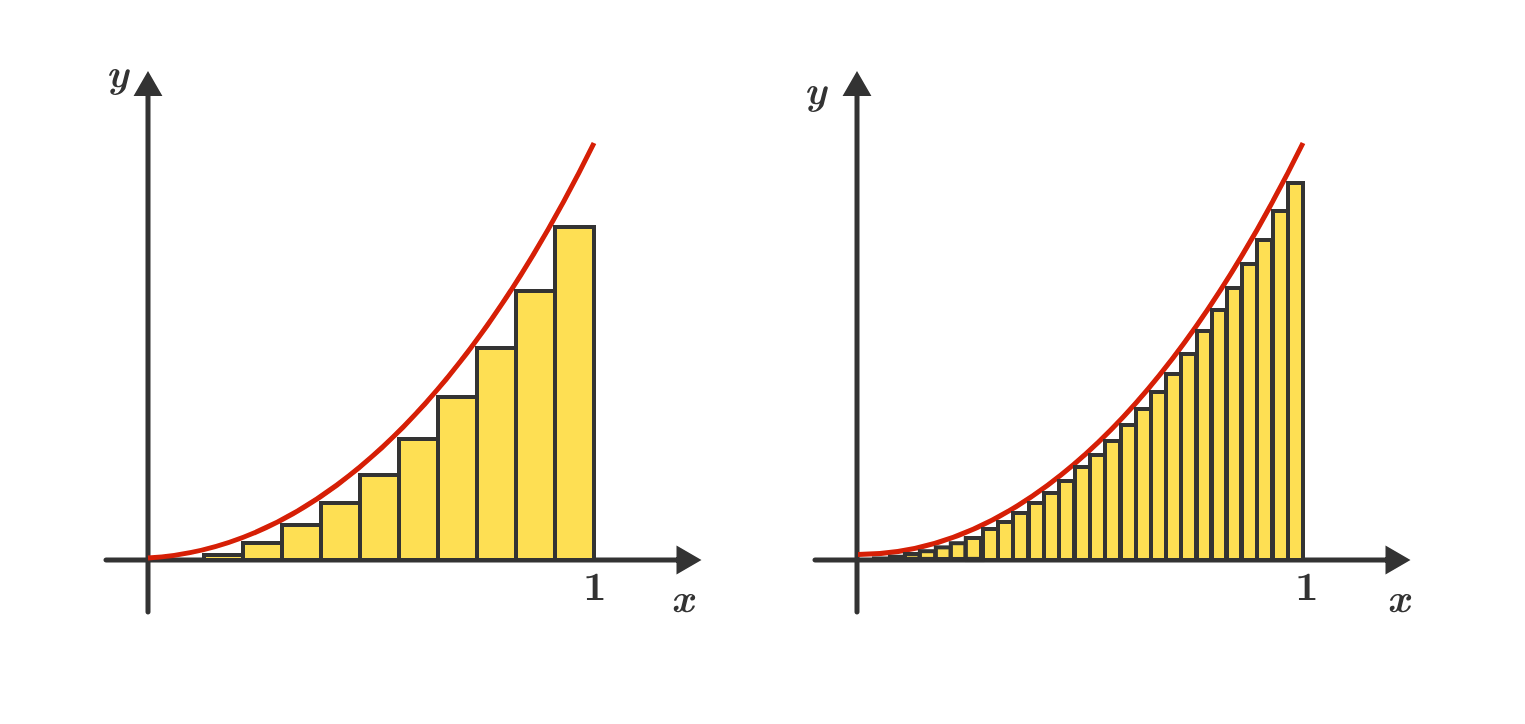This gives us a crude estimate for the area: we just add up the areas of each of the rectangles. The estimate gets better and better the more rectangles we use, as in the figure above (right).

In the limit where the number of rectangles becomes infinite, the sum gives us precisely the area we seek.

## Zeno's Paradox

### Introduction to Calculus

# Zeno's Paradox

To close out this unit, let's estimate the area below the parabola $$y = x^2,$$ above the $$x$$-axis, and between the lines $$x = 0$$ and $$x = 1.$$

Later in the course we'll find an estimate given by the sum $\text{Area} \approx \sum\limits_{j=1}^{n} \frac{j^2}{n^3},$ where $$n$$ is the number of rectangles. The coding environment below will compute this sum for you for any choice of $$n \geq 1$$ you like.

For very, very large $$n,$$ what is the best estimate for the area below the parabola?

import numpy as np

# initialize
n=2
A= 0.0
x = 1/n
j=0
# Begin Numerical Integration
while j <= n-1:
delta_A = x**2 * 1/n
x = x + 1/n
A = A + delta_A
j = j+1
print('Approximate Area Under the Curve =', A)
You need to be connected to run code

## Zeno's Paradox

### Introduction to Calculus

# Zeno's Paradox

We certainly have a long road ahead of us! But unlike Achilles, we're not in a race, and we should plan on enjoying the journey.

In the next unit, we'll continue our introduction to the basic ideas underlying our course by looking at one of its most important applications: computing the instantaneous rate of change of a function.

In trying to resolve Zeno's paradox of motion and the general area problem, we caught a glimpse of the foundational idea of the entire subject, the limit. When investigating rates of change, we'll also be unavoidably led to limits once more: limits form the unifying thread of this entire chapter.

## Zeno's Paradox

### Introduction to Calculus

×

Problem Loading...

Note Loading...

Set Loading...